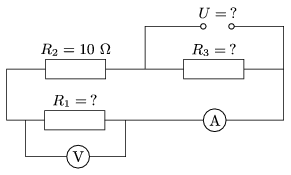Mathematical and Physical Journal
for High Schools
Issued by the MATFUND Foundation
 Already signed up? New to KöMaL?

#Problem P. 4024. (December 2007)

P. 4024. The power rating of resistor R3 shown in the figure is 15 W. The reading on the ammeter is 500 mA and the reading on the voltmeter is 10 V. Find the resistances of R1 and R3. What is the electromotive force of the supply?(3 pont)

Deadline expired on January 11, 2008.

### Statistics:

 217 students sent a solution. 3 points: 169 students. 2 points: 19 students. 1 point: 23 students. 0 point: 1 student. Unfair, not evaluated: 5 solutionss.

Problems in Physics of KöMaL, December 2007